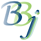# SQL — String Functions

The table below lists the string functions specified in the ODBC 3.51 and JDBC 2.0 specifications that are supported by the BASIS DBMS. The string functions are 1-based (the first character in the string is character 1).

Arguments denoted as string_exp can be the name of a column (of type CHAR), the result of another scalar function that returns a character string value, or a character-string literal.

Arguments denoted as start, length, or count can be a numeric literal or the result of another scalar function.

Function

Description

ASCII(string_exp)

Returns the ASCII code of the left-most character of string_exp as an integer.

CHAR(code)

Returns the character that has the ASCII code value returns the character specified by code. The code value should be between 0 and 255; otherwise, the return value is data source-dependent.

CONCAT(string_expl, string_exp2)

Returns a character string that is the result of concatenating string_exp2 to string_exp1. The resulting string is DBMS dependent. For example, if the column represented by string string_exp1 contains a NULL value, DB2 would return NULL, but SQL Server would return the non-NULL string.

DATEDIFF(date_exp1, date_exp2)

In BBj 12.0 and higher, returns the number of days between the specified dates by subtracting date_exp2 from date_exp1. If date_exp2 is later than date_exp1, the values returned will be negative.

DIFFERENCE(char,char)

Returns and integer value that indicates the difference between the values returned by the SOUNDEX function for string_exp1 and string_exp2.

INSERT(string_expl, start,length,string_exp2)

Returns a character string where length characters have been deleted from string_exp1 beginning at start and where string_exp2 has been inserted into string_exp1, beginning with start.

LCASE(string_exp)

Converts all upper case characters in string_exp to lower case.

LEFT(string_exp,count)

Returns the leftmost count characters of string_exp.

LENGTH(string_exp)

Returns the length of string_exp.

LEVENSHTEIN(string_exp1, string_exp2)

In BBj 9.0 and higher. From Wikipedia: "In information theory and computer science, the Levenshtein distance is a metric for measuring the amount of difference between two sequences (i.e. the so-called edit distance). The Levenshtein distance between two strings is given by the minimum number of operations neeeded to transform one string into the other, where an operation is an insertion, deletion, or substitution of a single character."

LOCATE(string_exp1, string_exp2[,start])

Returns the starting position of the first occurrence of string_exp1 within string_exp2. The search for the first occurrence of string_exp1 begins with the first character position in string_exp2, unless the optional start argument is specified. If start is specified, the search begins with the character position indicated by the value of start. The first character position string_exp2 is indicated by the value 1. If string_exp1 is not found within string_exp2, the value 0 is returned.

LTRIM(string_exp)

Returns the characters of string_exp, with leading blanks removed.

PARSE_TEMPLATE(template_string, stringbinary_exp)

In BBj 13.0 and higher, uses the string template specified in template_str to parse the character or binary data specified in stringbinary_exp and returns a human readable string containing each value from the template fields separated by commas.  For example, assume the column MY_COL in table MY_TABLE contains a value of length 7 for a record, and the bytes in that value are 65, 66, 67, 68, 69, 0, 5.  The following SQL statement returns a value of 'ABCDE', '5'

SELECT parse_template('COL1:C(5),COL2:I(2)', my_col) FROM my_table

The most common use for this function is to read the KEY data from a write auditing database to make the data more easily understood by those interested in the audit data.

REPEAT(string_exp,count)

Returns a character string composed of string_exp repeated count times.

REPLACE(string_exp1, string_exp2, string_exp3)

Replaces each occurrence of string_exp2 in string_exp1 with string_exp3.

RIGHT(string_exp,count)

Returns the last count characters of string_exp.

RTRIM(string_exp)

Returns the characters of string_exp with trailing blanks removed.

SHA2(string_exp[,bits])

In BBj 18.0 and higher, performs an SHA-2 one way hash on the specified string expression, its size being the number of bits specified. Valid bit values are 256, 384, and 512. If no value is specified for bits, the function defaults to 256. The value returned is a hexadecimal string containing the hashed value.

SOUNDEX(string_exp)

Returns a data source-dependent character string representing the sound of the words in string_exp. For example, SQL Server returns a four-digit SOUNDEX code.

SPACE(count)

Returns a character string consisting of count spaces.

STRIP_ACCENTS

In BBj 15.0 and higher, strips accents (~= diacritics) from a string. The case will be left unaltered. For example, 'à' will be replaced by 'a'. Note that ligatures will be left as is.

SUBSTRING(string_exp,start,length)

Returns a character string that is derived from string_exp beginning at the character position specified by start for length characters.

TRIM(string_exp)

Returns the characters of string_exp with leading and trailing blanks removed.

UCASE(string_exp)

Converts all lower-case characters in string_exp to upper case.

UUID()

In BBj 19.20 and higher, the UUID() function generates a universally unique identifier. The UUID is generated using a cryptographically strong pseudo-random number generator.

POS (stringA, relation, stringB [[,interval], [occurrence]])

Scans stringB for a substring with a specific relationship to stringA. This functions just like the POS() function, except that the relation parameter must be specified as a number as indicated in the following table:

Value Equivalent Relation Meaning
1 = Equal to
2 <>

Not equal to

3 <

Less than

4 <=

Less than or equal to

5 >

Greater than

6 >=

Greater than or equal to

## Example

Select RTRIM(last_name) + ' , ' + first_name from customer